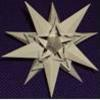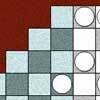# Search by Topic

#### Resources tagged with Powers & roots similar to Farey Neighbours:

Filter by: Content type:
Age range:
Challenge level:

### There are 37 results

Broad Topics > Numbers and the Number System > Powers & roots### How Many Solutions?

##### Age 16 to 18 Challenge Level:

Find all the solutions to the this equation.### Googol

##### Age 16 to 18 Short Challenge Level:

Find the smallest value for which a particular sequence is greater than a googol.### Em'power'ed

##### Age 16 to 18 Challenge Level:

Find the smallest numbers a, b, and c such that: a^2 = 2b^3 = 3c^5 What can you say about other solutions to this problem?### Negative Power

##### Age 14 to 16 Challenge Level:

What does this number mean ? Which order of 1, 2, 3 and 4 makes the highest value ? Which makes the lowest ?### Ab Surd Ity

##### Age 16 to 18 Challenge Level:

Find the value of sqrt(2+sqrt3)-sqrt(2-sqrt3)and then of cuberoot(2+sqrt5)+cuberoot(2-sqrt5).### In Between

##### Age 16 to 18 Challenge Level:

Can you find the solution to this algebraic inequality?### The Root of the Problem

##### Age 14 to 18 Challenge Level:

Find the sum of this series of surds.### Fibonacci Fashion

##### Age 16 to 18 Challenge Level:

What have Fibonacci numbers to do with solutions of the quadratic equation x^2 - x - 1 = 0 ?### Giants

##### Age 16 to 18 Challenge Level:

Which is the bigger, 9^10 or 10^9 ? Which is the bigger, 99^100 or 100^99 ?### Pythagorean Fibs

##### Age 16 to 18 Challenge Level:

What have Fibonacci numbers got to do with Pythagorean triples?### Roots Near 9

##### Age 14 to 16 Short Challenge Level:

For how many integers 𝑛 is the difference between √𝑛 and 9 is less than 1?### Perfectly Square

##### Age 14 to 16 Challenge Level:

The sums of the squares of three related numbers is also a perfect square - can you explain why?### Plus or Minus

##### Age 16 to 18 Challenge Level:

Make and prove a conjecture about the value of the product of the Fibonacci numbers $F_{n+1}F_{n-1}$.### Root to Poly

##### Age 14 to 16 Challenge Level:

Find the polynomial p(x) with integer coefficients such that one solution of the equation p(x)=0 is $1+\sqrt 2+\sqrt 3$.### Enriching Experience

##### Age 14 to 16 Challenge Level:

Find the five distinct digits N, R, I, C and H in the following nomogram### Rational Roots

##### Age 16 to 18 Challenge Level:

Given that a, b and c are natural numbers show that if sqrt a+sqrt b is rational then it is a natural number. Extend this to 3 variables.### Archimedes and Numerical Roots

##### Age 14 to 16 Challenge Level:

The problem is how did Archimedes calculate the lengths of the sides of the polygons which needed him to be able to calculate square roots?### Staircase

##### Age 16 to 18 Challenge Level:

Solving the equation x^3 = 3 is easy but what about solving equations with a 'staircase' of powers?### Square Pair Circles

##### Age 16 to 18 Challenge Level:

Investigate the number of points with integer coordinates on circles with centres at the origin for which the square of the radius is a power of 5.### Number Rules - OK

##### Age 14 to 16 Challenge Level:

Can you convince me of each of the following: If a square number is multiplied by a square number the product is ALWAYS a square number...### Rationals Between...

##### Age 14 to 16 Challenge Level:

What fractions can you find between the square roots of 65 and 67?### The Root Cause

##### Age 16 to 18 Challenge Level:

Prove that if a is a natural number and the square root of a is rational, then it is a square number (an integer n^2 for some integer n.)### Equal Temperament

##### Age 14 to 16 Challenge Level:

The scale on a piano does something clever : the ratio (interval) between any adjacent points on the scale is equal. If you play any note, twelve points higher will be exactly an octave on.### Fit for Photocopying

##### Age 14 to 16 Challenge Level:

Explore the relationships between different paper sizes.### Archimedes Numerical Roots

##### Age 16 to 18 Challenge Level:

How did Archimedes calculate the lengths of the sides of the polygons which needed him to be able to calculate square roots?### Consecutive Squares

##### Age 14 to 16 Challenge Level:

The squares of any 8 consecutive numbers can be arranged into two sets of four numbers with the same sum. True of false?### Rachel's Problem

##### Age 14 to 16 Challenge Level:

Is it true that $99^n$ has 2n digits and $999^n$ has 3n digits? Investigate!### Lost in Space

##### Age 14 to 16 Challenge Level:

How many ways are there to count 1 - 2 - 3 in the array of triangular numbers? What happens with larger arrays? Can you predict for any size array?### Mod 7

##### Age 16 to 18 Challenge Level:

Find the remainder when 3^{2001} is divided by 7.### Guesswork

##### Age 14 to 16 Challenge Level:

Ask a friend to choose a number between 1 and 63. By identifying which of the six cards contains the number they are thinking of it is easy to tell them what the number is.### Double Trouble

##### Age 14 to 16 Challenge Level:

Simple additions can lead to intriguing results...### Unusual Long Division - Square Roots Before Calculators

##### Age 14 to 16 Challenge Level:

However did we manage before calculators? Is there an efficient way to do a square root if you have to do the work yourself?### Power Countdown

##### Age 14 to 16 Challenge Level:

In this twist on the well-known Countdown numbers game, use your knowledge of Powers and Roots to make a target.### Function Pyramids

##### Age 16 to 18 Challenge Level:

A function pyramid is a structure where each entry in the pyramid is determined by the two entries below it. Can you figure out how the pyramid is generated?### Deep Roots

##### Age 14 to 16 Challenge Level:

Find integer solutions to: $\sqrt{a+b\sqrt{x}} + \sqrt{c+d.\sqrt{x}}=1$### Napier's Location Arithmetic

##### Age 14 to 16 Challenge Level:

Have you seen this way of doing multiplication ?### Route to Root

##### Age 16 to 18 Challenge Level:

A sequence of numbers x1, x2, x3, ... starts with x1 = 2, and, if you know any term xn, you can find the next term xn+1 using the formula: xn+1 = (xn + 3/xn)/2 . Calculate the first six terms of this. . . .